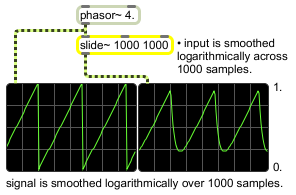# slide~

Filter a signal logarithmically

## Description

slide~ filters an input signal logarithmically between changes in signal value. The formula is y (n) = y (n-1) + ((x (n) - y (n-1))/slide). It's particularly useful for envelope following and lowpass filtering.

## Arguments

Name Type Opt Description
slide-up float opt Specifies the slide up value. The default is 1. (Also accepts integers for these arguments as well as the associated messages which address these parameter settings.)
slide-down float opt A second argument specifies the slide down value. The default is 1. (Also accepts integers for these arguments as well as the associated messages which address these parameter settings.)

## Messages

 int current-output-sample [int] Sets the current output sample value (the value from which the next incoming value will smoothly transition from). float current-output-sample [float] Sets the current output sample value (the value from which the next incoming value will smoothly transition from). (inlet1) slide-up [float] In middle inlet: Specifies the slide up value to be used when an incoming value is greater than the current value. (inlet2) slide-down [float] In right inlet: Specifies the slide down value to be used when an incoming value is less than the current value. signal A signal to be filtered. Whenever a new value is received, slide~ filters the input signal logarithmically between changes in signal value using the formula: y (n) = y (n-1) + ((x (n) - y (n-1))/slide) A given sample output from slide~ is equal to the last sample's value plus the difference between the last sample's value and the input divided by the slide value. Given a slide value of 1, the output will therefore always equal the input. Given a slide value of 10, the output will only change 1/10th as quickly as the input. This can be particularly useful for lowpass filtering or envelope following. slide_down slide-down [float] The message slide_down followed by a float will specify the slide-down value to be used when an incoming value is less than the current value. slide_up slide-up [float] The message slide_up followed by a float will specify the slide-up value to be used when an incoming value is greater than the current value. reset Sets the current output sample value to 0(the next incoming value will smoothly transition from that 0).

## Output

signal: The filtered signal.

## Examplesslide~ performs logarithmic smoothing of an input signal